Next: Navier-Stokes Equation Up: Mathematical Models of Fluid Previous: Convective Time Derivative

# Momentum Conservation

Consider a fixed volumesurrounded by a surface. The-component of the total linear momentum contained withinis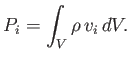(1.43)

Moreover, the flux of-momentum across, and out of, is [see Equation (1.29)]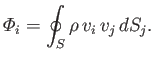(1.44)

Finally, the-component of the net force acting on the fluid withinis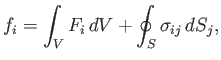(1.45)

where the first and second terms on the right-hand side are the contributions from volume and surface forces, respectively.

Momentum conservation requires that the rate of increase of the net-momentum of the fluid contained within, plus the flux of-momentum out of, is equal to the rate of-momentum generation within. Of course, from Newton's second law of motion, the latter quantity is equal to the-component of the net force acting on the fluid contained within. Thus, we obtain [cf., Equation (1.31)]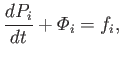(1.46)

which can be written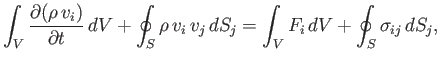(1.47)

because the volumeis non-time-varying. Making use of the tensor divergence theorem, this becomes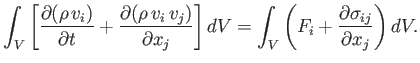(1.48)

However, the previous result is valid irrespective of the size, shape, or location of volume, which is only possible if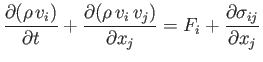(1.49)

everywhere inside the fluid. Expanding the derivatives, and rearranging, we obtain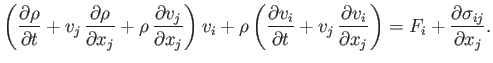(1.50)

In tensor notation, the continuity equation (1.37) is written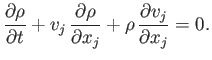(1.51)

So, combining Equations (1.50) and (1.51), we obtain the following fluid equation of motion,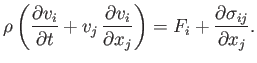(1.52)

An alternative form of this equation is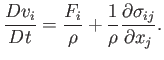(1.53)

The previous equation describes how the net volume and surface forces per unit mass acting on a co-moving fluid element determine its acceleration.Next: Navier-Stokes Equation Up: Mathematical Models of Fluid Previous: Convective Time Derivative
Richard Fitzpatrick 2016-03-31